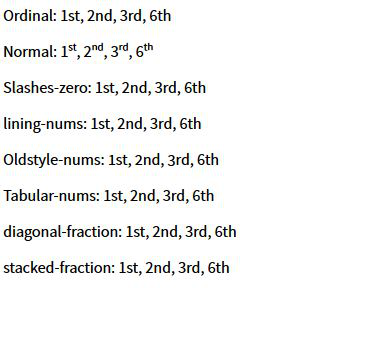# CSS font-variant-numeric Property

The font-variant-numeric property of CSS is used to control the usage of alternate glyphs. This is done in terms of units or markers such as numbers or fractions.

Syntax:

`font-variant-numeric: value`

Property values:

• normal: Using normal will remove each effect of font-variant-numeric property.
• ordinal: This value directly indicates the open type values i.e ordn. The term makes use of special glyphs for ordinal markers.
• slashed-zero: Slashed-zero used a zero with a slash. This property values prove to be very useful while distinguishing between 0 and O.
• lining-nums: Lining nums property corresponds to the open type values i.e lnum. This keyword activates the numbers lying on the baseline.
• oldstyle-nums: Oldstyle-nums property corresponds to the open type values i.e onum. This keyword activates the set of Figures where some numbers have descendent.
• proportional-nums: This property activates those norms where not every number is of same size. Its Open type value is pnum.
• tabular-nums: Tabular-nums open type value is tnum. It activates those set of figures where set of numbers is of the same size.
• diagonal-fractions: It open type value is frac. This activates those set of figures where numerator and denominator are made smaller and are separated by slash.
• stacked-fractions: It open type value is arac. This activates those set of figures where numerator and denominator are made smaller, stacked, and are separated by horizontal line.

Example:

 ` ` `<``html` `lang``=``"en"``> ` `    ``<``head``> ` `        ``<``meta` `charset``=``"UTF-8"` `/> ` `        ``<``meta` `name``=``"viewport"`  `              ``content="``width``=``device``-width, ` `                       ``initial-scale``=``1``.0" /> ` `        ``<``link` `            ``href= ` `"https://fonts.googleapis.com/css2?``family``=``Source``+Sans+Pro:ital, ` `              ``wght@0, 200;0, 400;1, 400&``display``=``swap``" ` `            ``rel``=``"stylesheet"` `        ``/> ` `        ``<``title``>Document ` `    `` ` `    ``<``style``> ` `        ``* { ` `            ``font-family: "Source Sans Pro"; ` `        ``} ` `        ``.value1 { ` `            ``font-variant-numeric: normal; ` `        ``} ` `        ``.value2 { ` `            ``font-variant-numeric: ordinal; ` `        ``} ` `        ``.value3 { ` `            ``font-variant-numeric: slashed-zero; ` `        ``} ` `        ``.value4 { ` `            ``font-variant-numeric: lining-nums; ` `        ``} ` `        ``.value5 { ` `            ``font-variant-numeric: oldstyle-nums; ` `        ``} ` `        ``.value6 { ` `            ``font-variant-numeric: tabular-nums; ` `        ``} ` `        ``.value7 { ` `            ``font-variant-numeric: diagonal-fractions; ` `        ``} ` `        ``.value7 { ` `            ``font-variant-numeric: stacked-fractions; ` `        ``} ` `    `` ` `    ``<``body``> ` `        ``<``p``> ` `            ``<``span``>Ordinal: ` `            ``<``span` `class``=``"value1"``>1st, 2nd, 3rd, 6th ` `        `` ` `        ``<``p``> ` `            ``<``span``>Normal: ` `            ``<``span` `class``=``"value2"``>1st, 2nd, 3rd, 6th ` `        `` ` `        ``<``p``> ` `            ``<``span``>Slashes-zero: ` `            ``<``span` `class``=``"value3"``>1st, 2nd, 3rd, 6th ` `        `` ` `        ``<``p` `class``=``"value4"``> ` `            ``<``span``>lining-nums: ` `            ``1st, 2nd, 3rd, 6th ` `        `` ` `        ``<``p` `class``=``"value5"``> ` `            ``<``span``>Oldstyle-nums: ` `            ``1st, 2nd, 3rd, 6th ` `        `` ` `        ``<``p` `class``=``"value6"``> ` `            ``<``span``>Tabular-nums: ` `            ``1st, 2nd, 3rd, 6th ` `        `` ` `        ``<``p` `class``=``"value7"``> ` `            ``<``span``>diagonal-fraction: ` `            ``1st, 2nd, 3rd, 6th ` `        `` ` `        ``<``p` `class``=``"value7"``> ` `            ``<``span``>stacked-fraction: ` `            ``1st, 2nd, 3rd, 6th ` `        `` ` `    `` ` ` `

Output:Supported Browsers:

• Edge
• Mozilla Firefox
• Opera
• SafariMy Personal Notes arrow_drop_upCheck out this Author's contributed articles.

If you like GeeksforGeeks and would like to contribute, you can also write an article using contribute.geeksforgeeks.org or mail your article to contribute@geeksforgeeks.org. See your article appearing on the GeeksforGeeks main page and help other Geeks.

Please Improve this article if you find anything incorrect by clicking on the "Improve Article" button below.

Article Tags :
Practice Tags :

Be the First to upvote.

Please write to us at contribute@geeksforgeeks.org to report any issue with the above content.